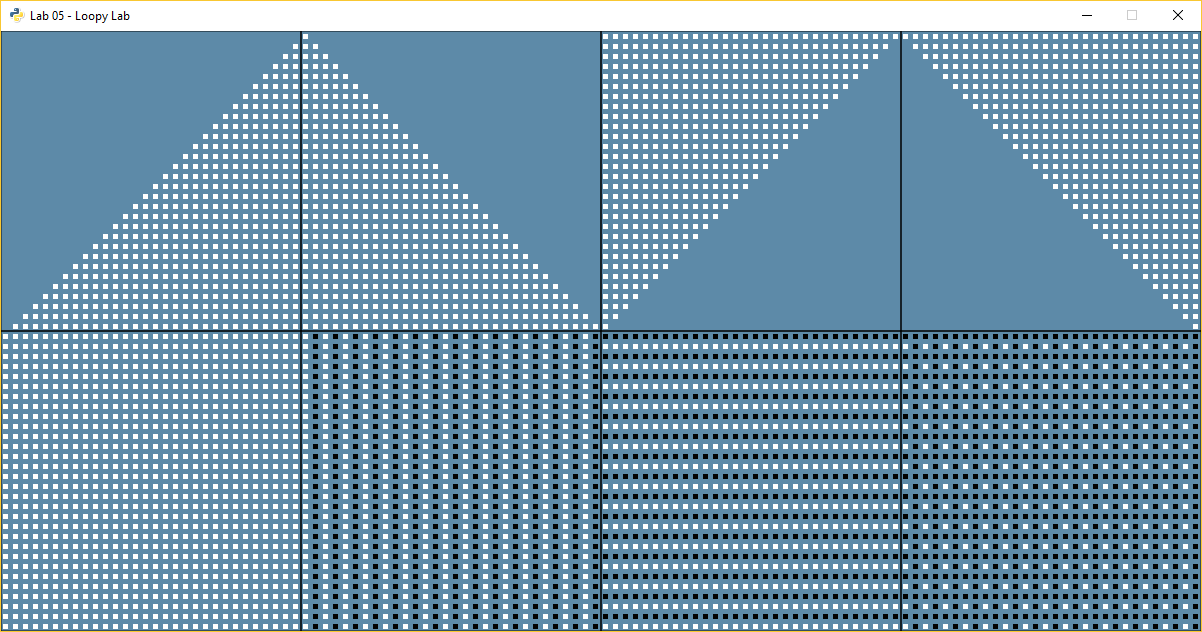# Lab 5: Loopy Lab

The goal of this lab is to practice using nested `for` loops. We will create a program that makes the following image:For sanity’s sake, let’s give a number to each section. Then when we ask questions we can say which section we are talking about. Do not put the numbers in the final program. Here are the section numbers:Great! All these items can be created using nested `for` loops.

## Getting Started

Below is some code to get you started. Underneath each of the comments, fill in the code required to make the pattern.

```import arcade

def draw_section_outlines():
# Draw squares on bottom

# Draw squares on top

def draw_section_1():
for row in range(30):
for column in range(30):
x = 0  # Instead of zero, calculate the proper x location using 'column'
y = 0  # Instead of zero, calculate the proper y location using 'row'

def draw_section_2():
# Below, replace "pass" with your code for the loop.
# Use the modulus operator and an if statement to select the color
# Don't loop from 30 to 60 to shift everything over, just add 300 to x.
pass

def draw_section_3():
# Use the modulus operator and an if/else statement to select the color.
# Don't use multiple 'if' statements.
pass

def draw_section_4():
# Use the modulus operator and just one 'if' statement to select the color.
pass

def draw_section_5():
# Do NOT use 'if' statements to complete 5-8. Manipulate the loops instead.
pass

def draw_section_6():
pass

def draw_section_7():
pass

def draw_section_8():
pass

def main():
# Create a window
arcade.open_window(1200, 600, "Lab 05 - Loopy Lab")

# Draw the outlines for the sections
draw_section_outlines()

# Draw the sections
draw_section_1()
draw_section_2()
draw_section_3()
draw_section_4()
draw_section_5()
draw_section_6()
draw_section_7()
draw_section_8()

main()
```

## Scoring

There are 20 possible points:

• Section 1: 2 pts

• Section 2: 2 pts

• Section 3: 2 pts

• Section 4: 2 pts

• Completing any section 1-4: 1 pt

• Section 5: 2 pts

• Section 6: 2 pts

• Section 7: 2 pts

• Section 8: 2 pts

• Completing any section 5-8: 1 pt

• Code Style: 2 pts (Should have no yellow lines on right side of PyCharm)

## Hints

• Each little square is a 5x5 pixel square.

• If the center of each square is 5 apart, you won’t see an edge and it will look like one big square.

• Remember, row controls up and down, so it corresponds to y. Column corresponds to x.

• When working on sections 2-8, you can simple add to the x and/or y values to shift everything over. For example, just add 300 to all the x values in section 2, to put it in the second box.

• Each section is 300x300 pixels.

• You will only need two `for` loops. Do not use a third nested `for` loop.

• Section 4 only needs one `if` statement. The trick is to use an `and`.

• Remember that the `%` sign is the modulus. It calculates the remainder. So:

```0 % 3 = 0
1 % 3 = 1
2 % 3 = 2
3 % 3 = 0
4 % 3 = 1
5 % 3 = 2
6 % 3 = 0
```
• You can count “backwards” by subtracting.

```print("Count up!")
for i in range(10):
print(i)

print()
print("Count down, even if i is going up")
for i in range(10):
x = 9 - i
print("i is", i, "and 9-i is", x)
```

This prints:

```Count up!
0
1
2
3
4
5
6
7
8
9

Count down, even if i is going up
i is 0 and 9-i is 9
i is 1 and 9-i is 8
i is 2 and 9-i is 7
i is 3 and 9-i is 6
i is 4 and 9-i is 5
i is 5 and 9-i is 4
i is 6 and 9-i is 3
i is 7 and 9-i is 2
i is 8 and 9-i is 1
i is 9 and 9-i is 0
```